Area of combination of figures : segment based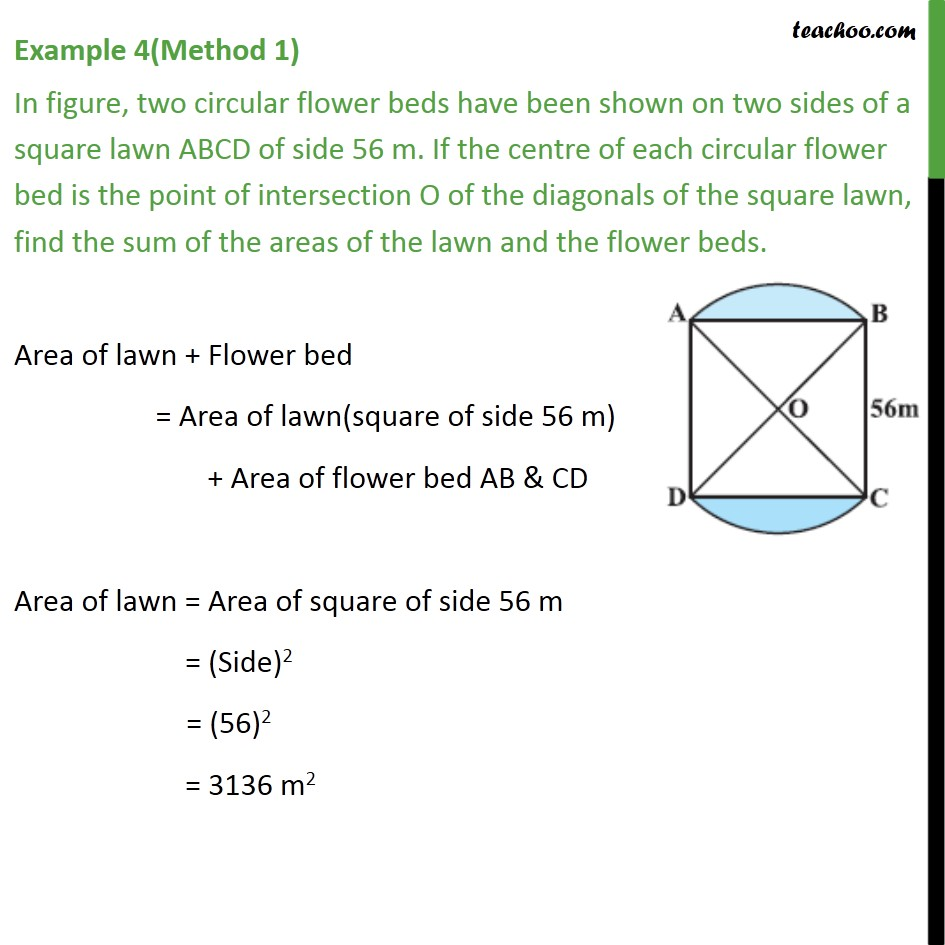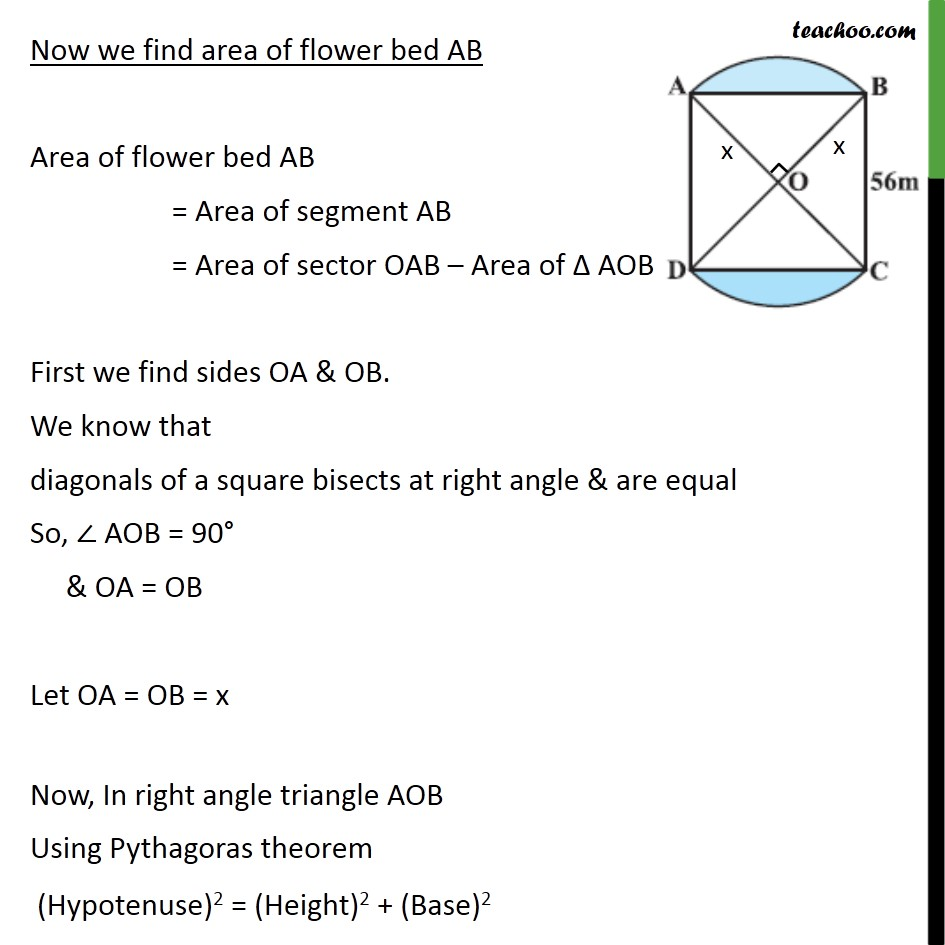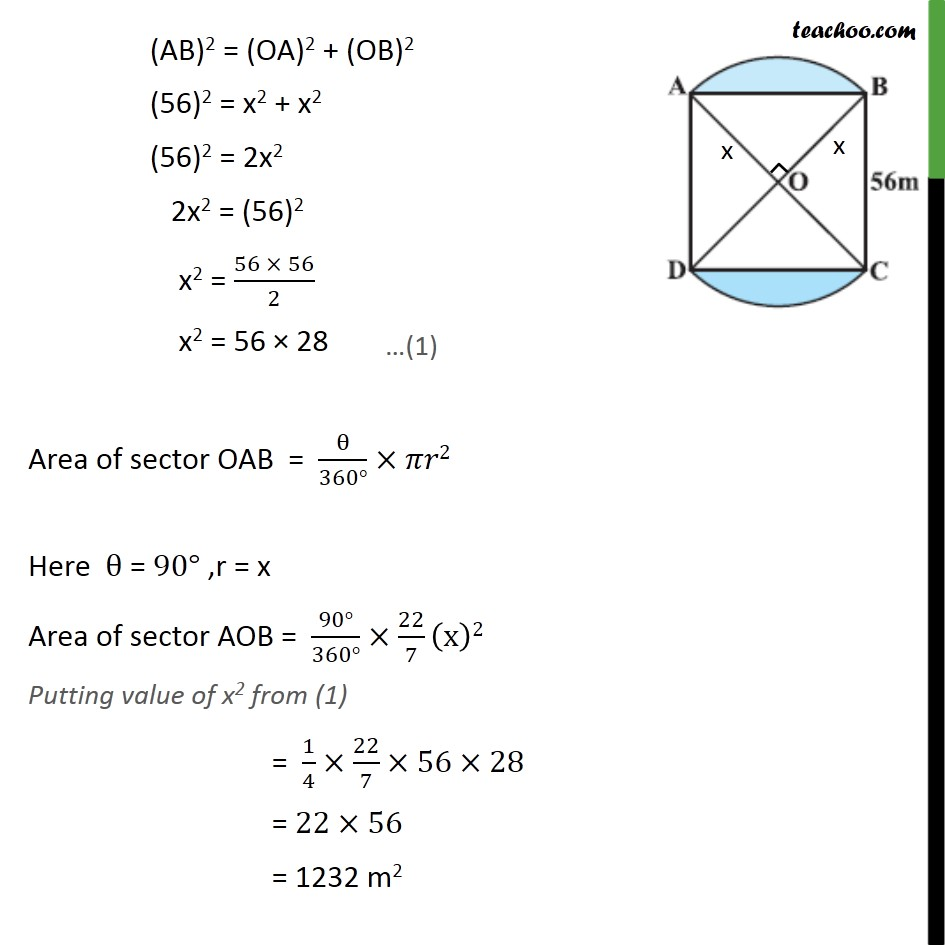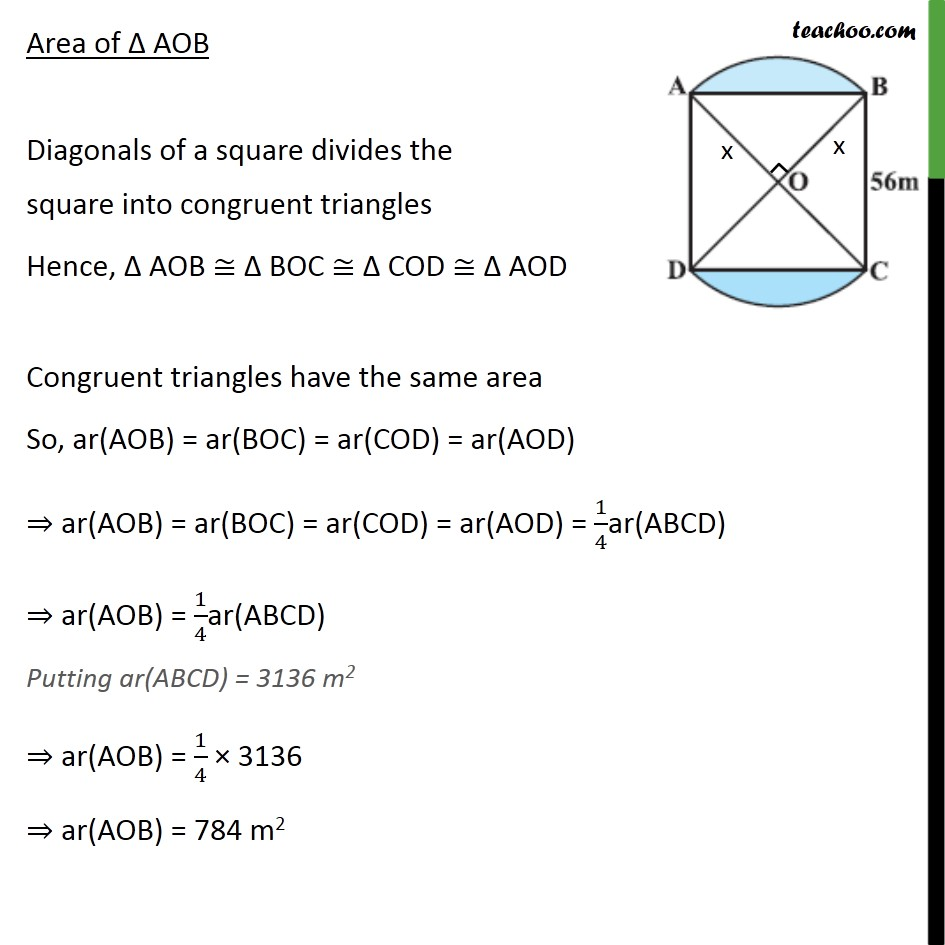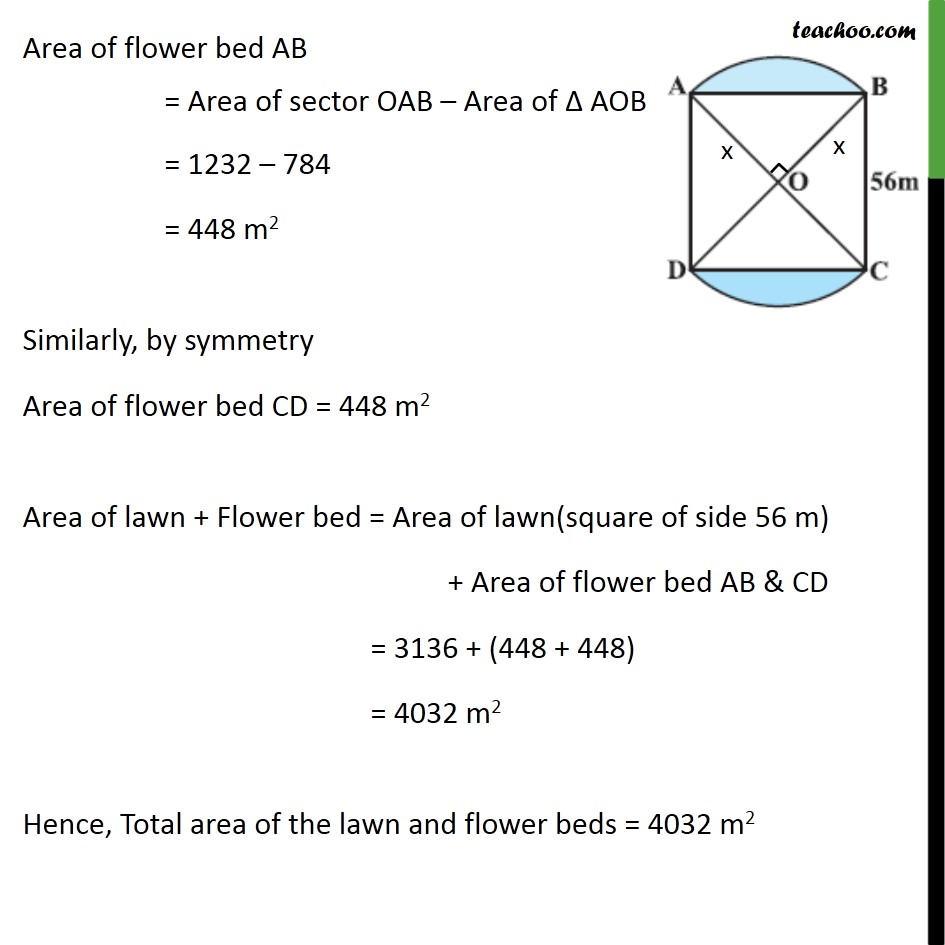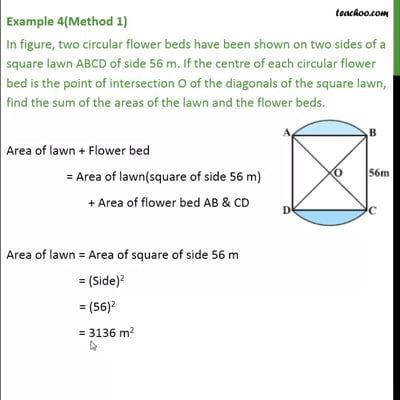This video is only available for Teachoo black users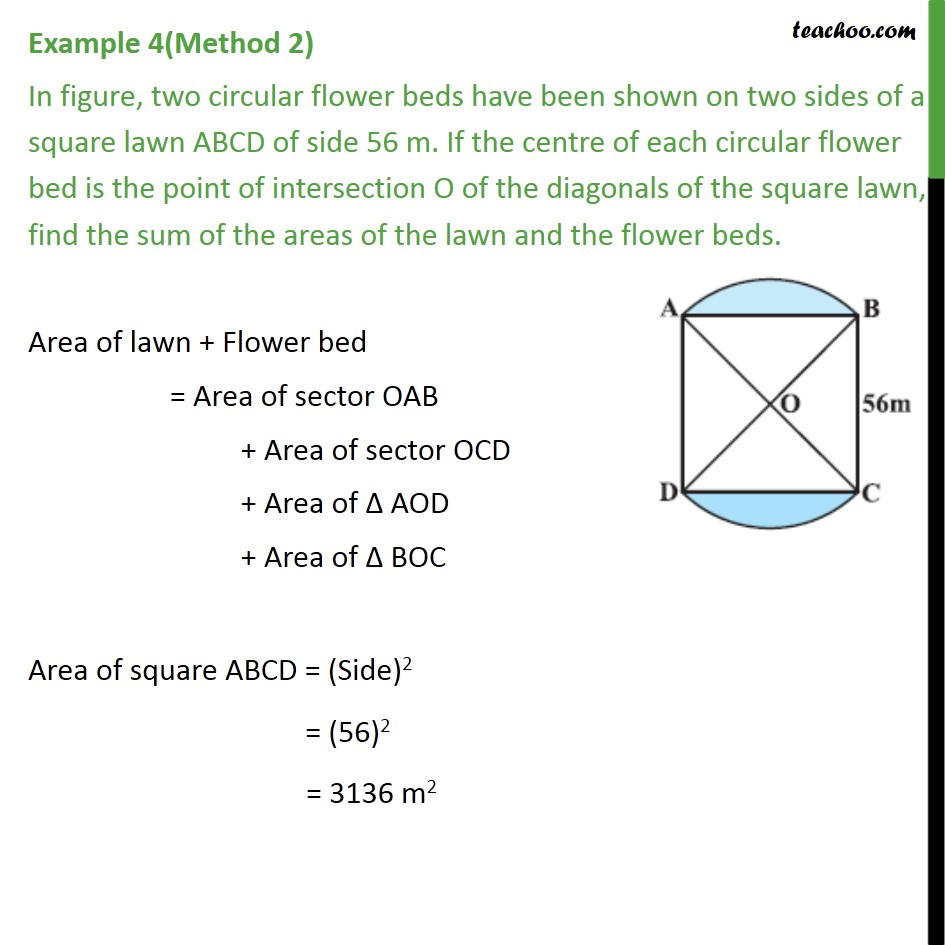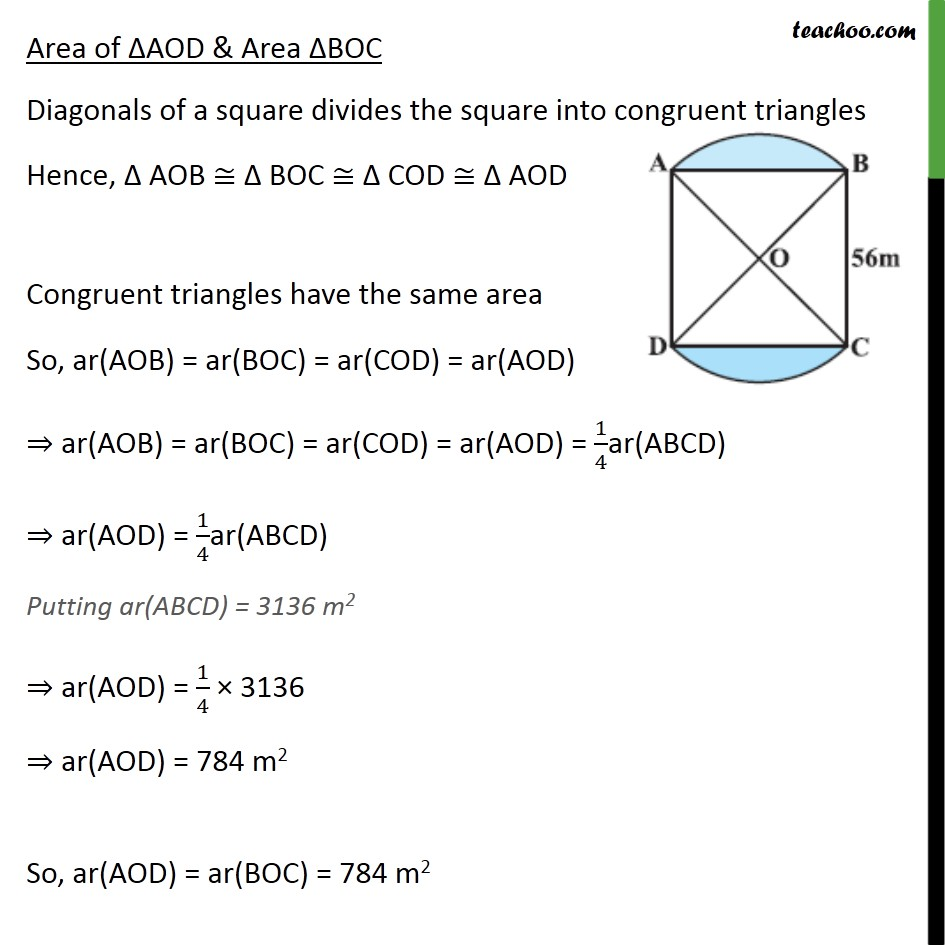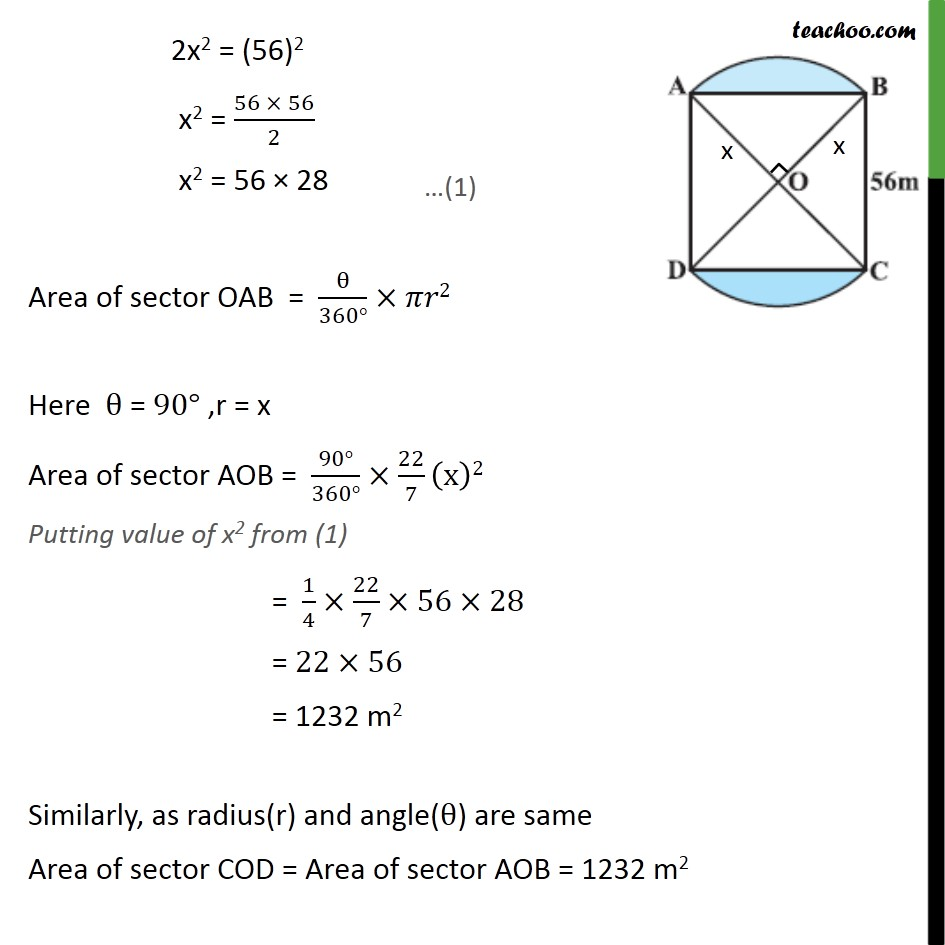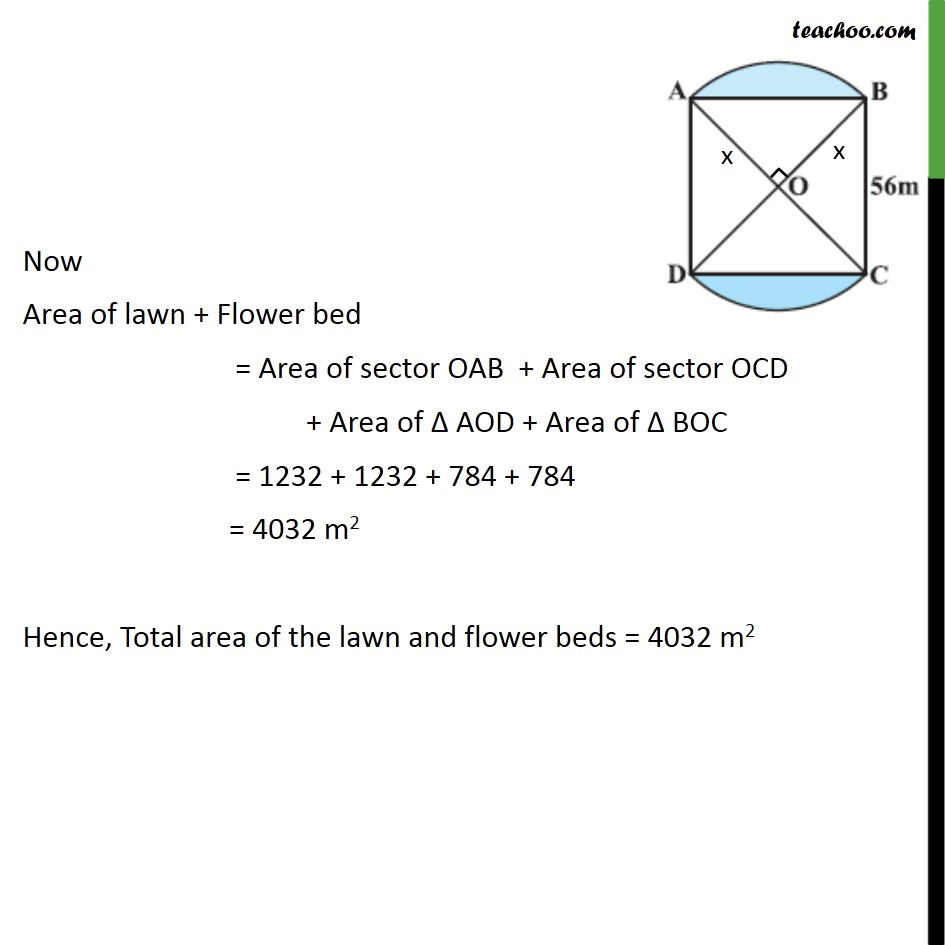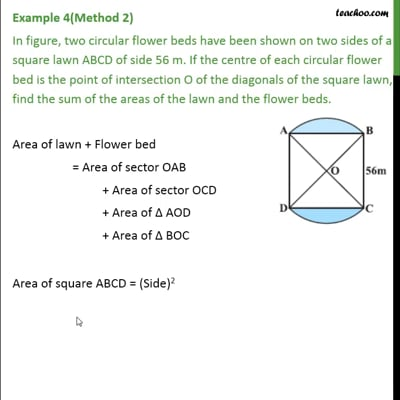This video is only available for Teachoo black users

Introducing your new favourite teacher - Teachoo Black, at only ₹83 per month

### Transcript

Example 4(Method 1) In figure, two circular flower beds have been shown on two sides of a square lawn ABCD of side 56 m. If the centre of each circular flower bed is the point of intersection O of the diagonals of the square lawn, find the sum of the areas of the lawn and the flower beds. Area of lawn + Flower bed = Area of lawn(square of side 56 m) + Area of flower bed AB & CD Area of lawn = Area of square of side 56 m = (Side)2 = (56)2 = 3136 m2 Now we find area of flower bed AB Area of flower bed AB = Area of segment AB = Area of sector OAB – Area of Δ AOB First we find sides OA & OB. We know that diagonals of a square bisects at right angle & are equal So, ∠ AOB = 90° & OA = OB Let OA = OB = x Now, In right angle triangle AOB Using Pythagoras theorem (Hypotenuse)2 = (Height)2 + (Base)2 (AB)2 = (OA)2 + (OB)2 (56)2 = x2 + x2 (56)2 = 2x2 2x2 = (56)2 x2 = (56 × 56)/2 x2 = 56 × 28 Area of sector OAB = θ/(360°)×𝜋𝑟2 Here θ = 90° ,r = x Area of sector AOB = (90°)/(360°)×22/7 (x)2 Putting value of x2 from (1) = 1/4×22/7×56×28 = 22×56 = 1232 m2 Area of Δ AOB Diagonals of a square divides the square into congruent triangles Hence, Δ AOB ≅ Δ BOC ≅ Δ COD ≅ Δ AOD Congruent triangles have the same area So, ar(AOB) = ar(BOC) = ar(COD) = ar(AOD) ⇒ ar(AOB) = ar(BOC) = ar(COD) = ar(AOD) = 1/4ar(ABCD) ⇒ ar(AOB) = 1/4ar(ABCD) Putting ar(ABCD) = 3136 m2 ⇒ ar(AOB) = 1/4 × 3136 ⇒ ar(AOB) = 784 m2 Area of flower bed AB = Area of sector OAB – Area of Δ AOB = 1232 – 784 = 448 m2 Similarly, by symmetry Area of flower bed CD = 448 m2 Area of lawn + Flower bed = Area of lawn(square of side 56 m) + Area of flower bed AB & CD = 3136 + (448 + 448) = 4032 m2 Hence, Total area of the lawn and flower beds = 4032 m2 Example 4(Method 2) In figure, two circular flower beds have been shown on two sides of a square lawn ABCD of side 56 m. If the centre of each circular flower bed is the point of intersection O of the diagonals of the square lawn, find the sum of the areas of the lawn and the flower beds. Area of lawn + Flower bed = Area of sector OAB + Area of sector OCD + Area of Δ AOD + Area of Δ BOC Area of square ABCD = (Side)2 = (56)2 = 3136 m2 Area of ΔAOD & Area ΔBOC Diagonals of a square divides the square into congruent triangles Hence, Δ AOB ≅ Δ BOC ≅ Δ COD ≅ Δ AOD Congruent triangles have the same area So, ar(AOB) = ar(BOC) = ar(COD) = ar(AOD) ⇒ ar(AOB) = ar(BOC) = ar(COD) = ar(AOD) = 1/4ar(ABCD) ⇒ ar(AOD) = 1/4ar(ABCD) Putting ar(ABCD) = 3136 m2 ⇒ ar(AOD) = 1/4 × 3136 ⇒ ar(AOD) = 784 m2 So, ar(AOD) = ar(BOC) = 784 m2 Now we find area of sector OAB First we find sides OA & OB. We know that diagonals of a square bisects at right angle and are equal So, ∠ AOB = 90° & OA = OB Let OA = OB = x Now, In right angle triangle AOB Using Pythagoras theorem (Hypotenuse)2 = (Height)2 + (Base)2 (AB)2 = (OA)2 + (OB)2 (56)2 = x2 + x2 (56)2 = 2x2 2x2 = (56)2 x2 = (56 × 56)/2 x2 = 56 × 28 Area of sector OAB = θ/(360°)×𝜋𝑟2 Here θ = 90° ,r = x Area of sector AOB = (90°)/(360°)×22/7 (x)2 Putting value of x2 from (1) = 1/4×22/7×56×28 = 22×56 = 1232 m2 Similarly, as radius(r) and angle(θ) are same Area of sector COD = Area of sector AOB = 1232 m2 Now Area of lawn + Flower bed = Area of sector OAB + Area of sector OCD + Area of Δ AOD + Area of Δ BOC = 1232 + 1232 + 784 + 784 = 4032 m2 Hence, Total area of the lawn and flower beds = 4032 m2